## Thursday, 7 August 2014

### chapter 6 - Important Remarks regarding Probability

Before moving on, some very important remarks need to be made:
• If events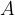$A$ and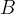$B$ are from two different sample spaces, then they are obviously independent. For eg, let us consider the experiment of throwing a coin and a die simultaneously. Any outcome of the coin throw is independent of any outcome on the die roll.
• Do not confuse mutually exclusive events with independent events! In fact,
If$A$ and$B$ are two non-impossible mutually exclusive events, then they are not independent.
Reason
This should be obvious. If$A$ and$B$ are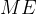$ME$ events, then the occurrence of one rules out, i.e, affects, the occurrence of the other which means that they are not independent. Mathematically speaking,
We have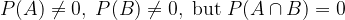$P(A) \ne 0,\;P(B) \ne 0,\;{\rm{but}}\;P(A \cap B) = 0$
be cause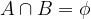$A \cap B = \phi$. Thus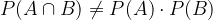$P(A \cap B) \ne P(A) \cdot P(B)$
If$A$ and$B$ are two non-impossible independent events, they they are not mutually exclusive.
Reason:
This is again very simple to understand. If$A$ and$B$ are independent events then obviously the occurrence of one does not rule out the occurrence of he other, which means that they are nor$ME$. Mathematically,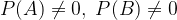$P(A) \ne 0,\;P(B) \ne 0$ Thus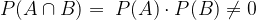$P(A \cap B) = \;P(A) \cdot P(B) \ne 0$ which means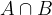$A \cap B$ is not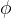$\phi$
Try to understand these two assertions with actual examples.
We now consider one final example before going on to the next section.
Scenario-5:
You are sitting in a class of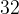$32$ students, and your eccentric Math Professor is teaching you probability. Suddenly, he pops this statement at the whole class:
“You know what! There is a more than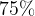$75\%$ chance that at least two students in this class share the same birthday!”
What would your reaction be? Would you term his assertion crazy? After all, the class has only$32$ students while a year has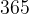$365$ days. That the chances of two students sharing the same birthday in such a small class could be greater than$75\%$ (which is pretty high!), will definitely strike one as strange.
However, it turns out that your professor is correct. Let us try to understand how. Later on, we’ll revisit this same problem with more rigor.
Considering that there is no time of the year when there is a sudden ‘baby boom’ where the rate of babies being born jumps abruptly, it is fair enough to assume that any random person you choose is equally likely to born on any day of the year. Thus, for example, each of the$32$ students in your class is equally likely to be born on any day of the year.
Let us first find the total number of ways in which these$32$ students could have been born during the year, or more precisely, the number of ways in which these$32$ students can be assigned birth-dates. This number is simply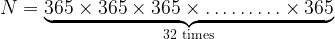$N = \underbrace {365 \times 365 \times 365 \times \ldots \ldots \ldots \times 365}_{32\,\,{\rm{times}}}$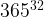${365^{32}}$
by the fundamental principle of counting since each student can be assigned a birth-date in$365$ ways. We now count the number of ways in which at least two students could share a birthday. However, you’ll appreciate that it will be easier to analyse the complementary case, namely finding the number of ways of assigning birth-dates to the students so that no two students share the same birthday. This number is simply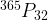${}^{365}{P_{32}}$, since from amongst$365$ birth-dates, we need to make unique assignments to the$32$ students. Thus, the probability of no two students sharing the same birthday will be$\dfrac{\overbrace{{{}^{365}{P_{32}}} }^{\rm{Comment:1}} } {{ \underbrace{{{(365)}^{32}}}_{\rm{Comment:2}} }}$ Comment:1 No. of assignment in which no birthdays are shared Comment:2 Total number of assignments of birth-dates
which equals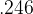$.246$.
Thus, the probability of the complementary event, i.e. of at least two students sharing a birthday, will be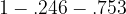$1 - .246 - .753$ or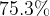$75.3\%$ Surprising!